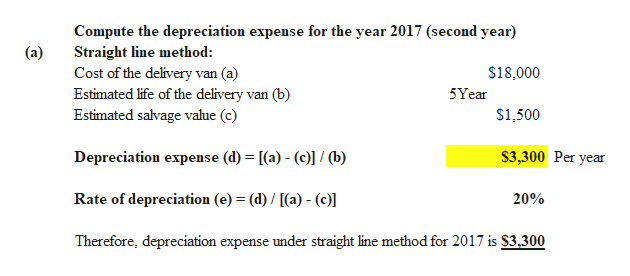# M8-12 Computing Depreciation Under Straight-Line and Double-Declining-BalanceA delivery van costing \$18,000 is expected to have a \$1,500 salvage value at the end of its useful life of 5years. Assume that the truck was purchased on January 1, 2016. Compute the depreciation expense for2017 (its second year) under each of the following depreciation methods:a. Straight-lineb. Double-declining-balance

Question
35 views
M8-12 Computing Depreciation Under Straight-Line and Double-Declining-Balance
A delivery van costing \$18,000 is expected to have a \$1,500 salvage value at the end of its useful life of 5
years. Assume that the truck was purchased on January 1, 2016. Compute the depreciation expense for
2017 (its second year) under each of the following depreciation methods:
a. Straight-line
b. Double-declining-balance
check_circle

Step 1help_outlineImage TranscriptioncloseCompute the depreciation expense for the year 2017 (second year) Straight line method: Cost of the delivery van (a) Estimated life of the delivery van (b) Estimated salvage value (c) (a) \$18,000 5Year \$1,500 Depreciation expense (d) = [(a) - (c)] / (b) \$3,300 Per year Rate of depreciation (e) = (d) / [(a) - (c) 20% Therefore, depreciation expense under straight line method for 2017 is \$3,300 fullscreen

### Want to see the full answer?

See Solution

#### Want to see this answer and more?

Solutions are written by subject experts who are available 24/7. Questions are typically answered within 1 hour.*

See Solution
*Response times may vary by subject and question.
Tagged in